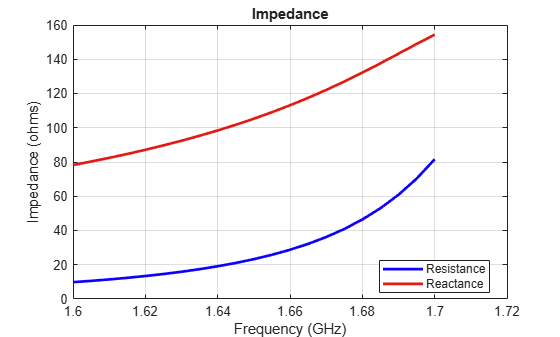# Model Infinite Ground Plane for Unbalanced Antennas

This example shows how to model an infinite ground plane and calculate fundamental antenna parameters for unbalanced antennas.

### Create Antenna on Infinite Ground Plane

Create a patch microstrip antenna on a ground plane of infinite length.

`p = patchMicrostrip('GroundPlaneLength', inf)`
```p = patchMicrostrip with properties: Length: 0.0750 Width: 0.0375 Height: 0.0060 Substrate: [1x1 dielectric] GroundPlaneLength: Inf GroundPlaneWidth: 0.0750 PatchCenterOffset: [0 0] FeedOffset: [-0.0187 0] Conductor: [1x1 metal] Tilt: 0 TiltAxis: [1 0 0] Load: [1x1 lumpedElement] ```

### View Antenna Geometry

View the physical construction of the patch microstrip antenna using infinite ground plane.

```figure; show(p);```### Calculate Impedance of Antenna

Calculate the impedance of the antenna over a frequency range of 1.60 GHz to 1.70 GHz.

```figure; impedance(p,1.60e9:5e6:1.70e9);```### Plot Radiation Pattern of Antenna

Plot the radiation pattern of the antenna at a frequency of 1.67 GHz.

```figure; pattern(p,1.67e9);```### Visualize Antenna Mesh

Mesh and view the infinite ground plane patch microstrip antenna.

```figure; mesh(p);```